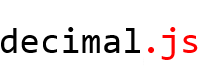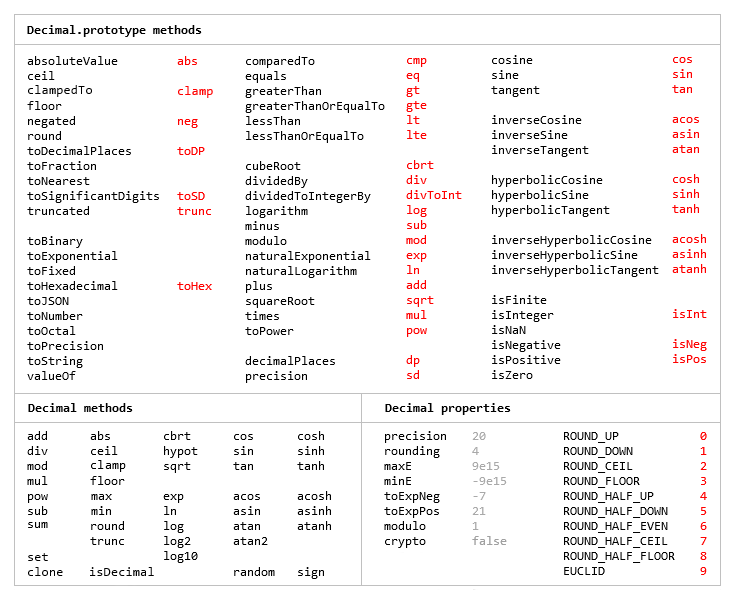Nutrias Punching Marmots

# npm

## decimal.js3.0.0 • Public • PublishedAn arbitrary-precision Decimal type for JavaScript.

## Features

• Faster, smaller, and perhaps easier to use than JavaScript versions of Java's BigDecimal
• Simple API but full-featured
• Replicates the `toExponential`, `toFixed`, `toPrecision` and `toString` methods of JavaScript's Number type
• Includes a `toFraction` and correctly-rounded `exp`, `ln`, `log` and `sqrt` functions
• Supports non-integer powers
• Works with numbers with or without fraction digits in bases from 2 to 64 inclusive
• Stores values in an accessible decimal floating-point format
• No dependencies
• Wide platform compatibility: uses JavaScript 1.5 (ECMAScript 3) features only
• Comprehensive documentation and test set
• 8 KB minified and gzippedThe library is similar to bignumber.js, but here precision is specified in terms of significant digits instead of decimal places, and all calculations are rounded to the precision (similar to Python's decimal module) rather than just those involving division.

This library also adds `exp`, `ln` and `log` functions, among others, and supports non-integer powers.

Another major difference is that this library enables multiple Decimal constructors to be created each with their own configuration (e.g. precision and range). This is, however, a significantly larger library than bignumber.js and the even smaller big.js.

The library is the single JavaScript file decimal.js (or minified, decimal.min.js).

It can be loaded via a script tag in an HTML document for the browser

``````<script src='./relative/path/to/decimal.js'></script>
``````

or as a CommonJS, Node.js or AMD module using `require`.

For Node, the library is also available from the npm registry

``````\$ npm install decimal.js
``````

``````require(['decimal'], function(Decimal) {
// Use Decimal here in local scope. No global Decimal.
});
``````

## Use

In all examples below, `var`, semicolons and `toString` calls are not shown. If a commented-out value is in quotes it means `toString` has been called on the preceding expression.

The library exports a single function object, `Decimal`, the constructor of Decimal numbers.

It accepts a value of type number (up to 15 significant digits only), string or Decimal.

``````x = new Decimal(123.4567)
y = new Decimal('123456.7e-3')
z = new Decimal(x)
x.equals(y) && y.equals(z) && x.equals(z)        // true
``````

A base from 2 to 36 inclusive can also be specified.

``````x = new Decimal(1011, 2)             // '11'
y = new Decimal('zz.9', 36)          // '1295.25'
z = x.plus(y)                        // '1306.25'
``````

A Decimal is immutable in the sense that it is not changed by its methods.

``````0.3 - 0.1                     // 0.19999999999999998
x = new Decimal(0.3)
x.minus(0.1)                  // '0.2'
x                             // '0.3'
``````

The methods that return a Decimal can be chained.

``````x.dividedBy(y).plus(z).times(9).floor()
x.times('1.23456780123456789e+9').plus(9876.5432321).dividedBy('4444562598.111772').ceil()
``````

Many method names have a shorter alias.

``````x.squareRoot().dividedBy(y).toPower(3).equals(x.sqrt().div(y).pow(3))         // true
x.cmp(y.mod(z).neg()) == 1 && x.comparedTo(y.modulo(z).negated()) == 1        // true
``````

Like JavaScript's Number type, there are `toExponential`, `toFixed` and `toPrecision` methods

``````x = new Decimal(255.5)
x.toExponential(5)              // '2.55500e+2'
x.toFixed(5)                    // '255.50000'
x.toPrecision(5)                // '255.50'
``````

and a base can be specified for `toString`.

``````x.toString(16)        // 'ff.8'
``````

There is a `toFraction` method with an optional maximum denominator argument

``````y = new Decimal(355)
pi = y.dividedBy(113)        // '3.1415929204'
pi.toFraction()              // [ '7853982301', '2500000000' ]
pi.toFraction(1000)          // [ '355', '113' ]
``````

and `isNaN` and `isFinite` methods, as `NaN` and `Infinity` are valid `Decimal` values.

``````x = new Decimal(NaN)                                           // 'NaN'
y = new Decimal(Infinity)                                      // 'Infinity'
x.isNaN() && !y.isNaN() && !x.isFinite() && !y.isFinite()      // true
``````

All calculations are rounded to the number of significant digits specified by the `precision` property of the Decimal constructor and rounded using the rounding mode specified by the `rounding` property.

As mentioned above, multiple Decimal constructors can be created, each with their own independent configuration which applies to all Decimal numbers created from it.

``````Decimal.config({ precision: 5, rounding: 4 })

// constructor is a factory method and it can also accept a configuration object
Decimal10 = Decimal.constructor({ precision: 10, rounding: 1 })

x = new Decimal(5)
y = new Decimal10(5)

x.div(3)                           // '1.6667'
y.div(3)                           // '1.666666666'

Decimal.precision                  // 5
Decimal10.precision                // 10
``````

Many of the methods of JavaScript's Math object are also replicated

``````Decimal.sqrt('6.98372465832e+9823')      // '8.3568682281821340204e+4911'
Decimal.pow(2, 0.0979843)                // '1.0702770511687781839'
``````

The value of a Decimal is stored in a floating point format in terms of a coefficient, exponent and sign.

``````x = new Decimal(-12345.67);
x.c                            // [ 12345, 6700000 ]    coefficient (base 10000)
x.e                            // 4                     exponent (base 10)
x.s                            // -1                    sign
``````

For further information see the API reference in the doc directory.

## Test

The test directory contains the test scripts for each method.

The tests can be run with Node or a browser.

To test a single method use, from a command-line shell in the root directory, for example

``````\$ node test/toFraction
``````

To test all the methods

``````\$ node test/every-test
``````

or

``````\$ npm test
``````

For the browser, see single-test.html and every-test.html in the test/browser directory,

## Build

For Node, if uglify-js is installed

``````npm install uglify-js -g
``````

then

``````npm run build
``````

will create decimal.min.js.

The decimal.min.js already present was created with Microsoft Ajax Minifier 5.8.

## Feedback

Open an issue, or email Michael M8ch88l@gmail.com

Thank you

MIT Expat.

See LICENCE.

## Change Log

#### 3.0.0

• 4/06/2014 `random` simplified. Major internal changes mean the properties of a Decimal must now be considered read-only.

#### 2.1.0

• 4/06/2014 Amend UMD

#### 2.0.3

• 8/05/2014 Fix NaN toNumber

#### 2.0.1

• 10/04/2014 Update npmignore

#### 2.0.0

• 10/04/2014 Add `toSignificantDigits`
• Remove `toInteger`
• No arguments to `ceil`, `floor`, `round` and `trunc`

#### 1.0.1

• 07/04/2014 Minor documentation clean-up

#### 1.0.0

• 02/04/2014 Initial release

## Keywords

### Install

`npm i decimal.js@3.0.0`

### Repository

github.com/MikeMcl/decimal.js